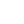----------- Your trusted source for independent sensor data- Photons to Photos------------ Last revised: 2018-02-26 15:00 GMT-5

# ------------------------------------- Optics Primer - Distance

--------------------------------------------------------- By Bill Claff

Now that we have the cardinal points here is how subject distance, focal  length, and magnification relate to each other:
S = ( 1 / m + 1 ) * f + i + ( 1 + m ) * f
where S is subject distance from the object plane to the image plane, f is focal length, m is (positive) magnification, and i is the inter-nodal distance.
Written in this form the term to the left of i is the distance in object space and the term to the right of i is the distance in image space.

Remember, in our diagrams the object and image planes are marked with "O" and "I" respectively.

We could apply this to our earlier example of the lens at 1/30th magnification:The distance from O to F is 1 / m * f ; 1548.30mm
The distance from F to H is 1 * f; 51.61mm
The distance from H to H' is i ; -12.20mm
The distance from H' to F' is 1 * f ; 51.61mm
The distance from F' to I is m * f ; 1.72mm

The total distance S, from O to I is 1641.04mm

Due to the slight paraxial variations the Optical Bench reports slightly different O to F and F' to I values for a total of 1643.04mm; quite close to the theoretical values.

It's interesting to note the symmetry between 1 / m and m in the distance formula. S is the same at 0.5x magnification and 2x magnification!
It's also clear than the minimum value of S is where magnification is 1.

Inter-nodal distance is not often known and is not relevant at longer subject distances so the formula is often simplified to:
S = ( 1 / m + 1 ) * f + ( 1 + m ) * f
which further simplifies to:
S = ( 1 + m )2 / m * f
This form is a common approximation but it obscures the foundation that I outlined above.

Finally, we arrive at a common grosser approximation when m is much smaller than 1:
S = f / m